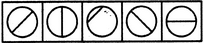# Non Verbal Reasoning - Classification - Discussion

### Discussion :: Classification - Section 1 (Q.No.15)

In each problem, out of the five figures marked (1), (2), (3), (4) and (5), four are similar in a certain manner. However, one figure is not like the other four. Choose the figure which is different from the rest.

15.

Choose the figure which is different from the rest.(1)     (2)     (3)     (4)     (5)

 [A]. 1 [B]. 2 [C]. 3 [D]. 4 [E]. 5

Explanation:

Only in fig. (3), the line segment is not a diameter of the circle.

 Roshan Roy said: (Mar 10, 2016) In figures (1),(2),(4),(5) the circles are divided into two equal semicircles or divided into half. But in figure (3) the circle is not divided into two equal semicircles or divided into half. So the answer is [C]. 3.